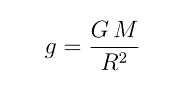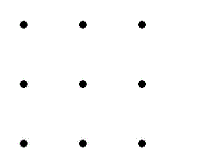# Recent Stinger Questions

Math Stinger #143

Consider the following sequence of numbers:

4, 6, 12, 18, 30, 42, 60, 72, 102, 108, ...

What are the next three numbers in the sequence?

Math Stinger #142

The acceleration due to Earth's gravity at the surface of the Earth iswhere G is the universal gravitational constant, M is the Earth's mass, and R is the Earth's radius.

(a) Develop the Taylor expansion through the second order in the small quantity h/R in order to find the accerlation due to gravity at an altitude h (assuming h << R) .

(b) How much smaller is g at the top of Stone Mountain than it is at sea level?  Find the difference in meters per second squared. For this part, use g = 9.81 m/ s^2 (at sea level), R = 6,371 km, and h = 514 m (which is approximately 1,683 ft).

Hint: for part (b), if the question was with regards to Mt. Everest (where h = 8,848 km), the answer would have been - 0.0272 (m/ s^2).

Math Stinger #141

Decipher the following simple-substitution cryptogram, in which every letter of the message is represented by a number.

8 23 9 26 4 16 24 26 25          8 18          22 24 13 22 7 13 22 8          8 18 19 7

Math Stinger #140

Here's a math question:

Find a four-digit number and a three-digit number such that: the first two digits of the four-digit number are a perfect square, as are the middle two digits and the last two digits. The three-digit number is a perfect cube and is the product of the four digits in the four-digit number.

Here's a logic question:

Connect all the dots using only 4 line segments without lifting your pen/pencil.